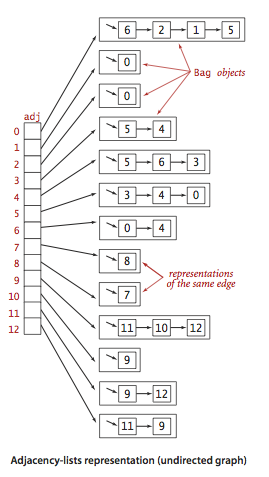# 14. 无向图

Wafer Li ... 2016-10-14 《Algorithm 第四版》笔记
• Algorithm
• 读书笔记

## # 14.1 术语

1. 相邻：如果两个顶点被至少一条边连接，那么就称顶点相邻，并称边依附于顶点。

2. 顶点的度：依附于它的边的条数

3. 子图：一幅图的子集（包括边和顶点）组成的图

4. 路径：由边顺序连接的一组顶点

其中又分为简单路径简单路径：没有重复顶点的路径 简单环：起点和终点必须相同的没有重复顶点和边的环

1. 连通图：如果从任何一个顶点都存在一条路径到达另一个任意节点，那么称这幅图为连通图

如果一副非联通图由若干个连通部分组成，那么这些部分都叫做极大连通子图

2. 无环图：就是没有环的图

树是一幅无环连通图

3. 密度：已经连接的顶点对占所有可能被连接的顶点对的比例。

这派生出了两个概念，稀疏图稠密图。 一般来说，如果一幅图中不同的边的数量在顶点总数$V$的一个小常数倍内，那么这幅图就是稀疏的

4. 二分图：一种能够将所有顶点分成两部分的图，其中每条边都连着两个不同的顶点

## # 14.2 表示法

### # 14.2.0 API

public class Graph {
public Graph(int V) // Create graph using the Vertex number

public Graph(In in) // Create graph from input stream

public int V();      // The number of Vertex

public int E();      // The number of Edge

// Add edge v-w into graph
public void addEdge(int v, int w);

// The vertexes adjacent to v

public String toString(); // The string explanation of graph
}

1
2
3
4
5
6
7
8
9
10
11
12
13
14
15
16
17

### # 14.2.3 邻接表数组1. 使用的空间和 $V + E$ 成正比
2. 添加一条边所需的时间为常数
3. 遍历顶点 v 的所有相邻顶点所需要的时间和 v 的度数成正比

public class Graph {
private final int V;        // Vertex number
private int E;              // Edge number

public Graph(int V) {
this.V = V;
this.E = 0;
for (int v = 0; v < V; v++) {
}
}

public Graph(In in) {
for (int i = 0; i < E; i++) {
}
}

public int V() {
return V;
}

public int E() {
return E;
}

public void addEdge(int v, int w) {
}

}
}

1
2
3
4
5
6
7
8
9
10
11
12
13
14
15
16
17
18
19
20
21
22
23
24
25
26
27
28
29
30
31
32
33
34
35
36
37
38
39
40
41
42